## Summary

Another cool effect is to make the surface disappear when it’s beyond a certain plane.

To follow this tutorial, it’s best to know how surface shaders work - you can find a tutorial how they work here.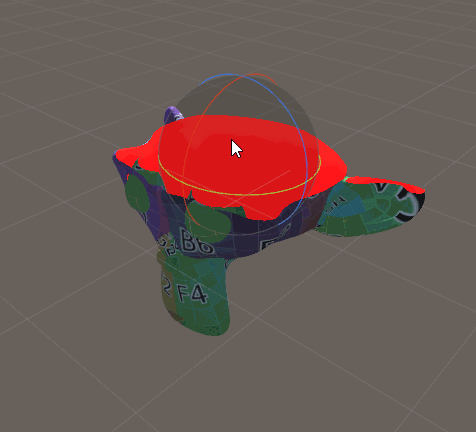## Define Plane

We start by creating a new C# script which will define the plane we use later and pass it to the shader. It has a material as a public variable which we will pass the plane to.

In the Update Method we create a new variable of the type Plane which unity already has. We pass it the the normal of the plane and a point on the plane. We will use the up vector of the transform the script is on as the normal and the position of the transform as the point on the plane.

Then we create a new 4d vector and put the normal of the new plane in the first three components and the distance from the origin in the 4th component. I’ll explain later how we’ll use those values.

Then we pass this new vector to the shader so we can use it there.

``````using System.Collections;
using System.Collections.Generic;
using UnityEngine;

public class ClippingPlane : MonoBehaviour {
//material we pass the values to
public Material mat;

//execute every frame
void Update () {
//create plane
Plane plane = new Plane(transform.up, transform.position);
//transfer values from plane to vector4
Vector4 planeRepresentation = new Vector4(plane.normal.x, plane.normal.y, plane.normal.z, plane.distance);
mat.SetVector("_Plane", planeRepresentation);
}
}
``````

To set up this script we add it to a empty gameobject and apply our material to the corresponding variable.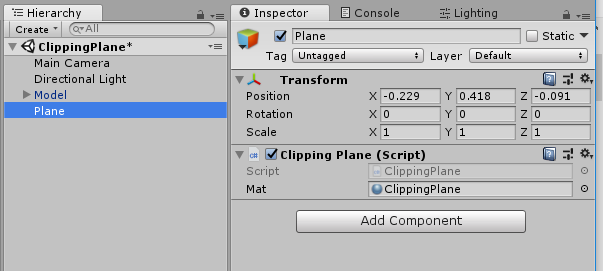## Clip Plane

Then we’ll write the shader. As a base for it we use the basic surface shader from this (tutorial](/2018/03/30/simple-surface.html).

First we add the plane variable we just passed into the material. Because we won’t write to it from the inspector, we don’t need a property for it.

``````float4 _Plane;
``````

In the surface shader we can then calculate the distance of the surface point to the plane if it was in the origin of the world. We do this by calculating the dot product between the surface point and the plane normal. For all points on that plane the dot product will return 0 because the position vector is orthogonal to the normal. For points that are above the plane the values will be positive because the vectors point in the same direction and for the surface points below the plane the dot product will be negative because they point away from the normal.

To do this comparison we need the world position, so we add it to our input struct. Then we get the dot product and just write it to the emission for now.

``````//input struct which is automatically filled by unity
struct Input {
float2 uv_MainTex;
float3 worldPos;
};
``````
``````//the surface shader function which sets parameters the lighting function then uses
void surf (Input i, inout SurfaceOutputStandard o) {
//calculate signed distance to plane
float distance = dot(i.worldPos, _Plane.xyz);
o.Emission = distance;
}
``````When we now rotate our plane object we can see the distance being calculated correctly, but it completely ignores the position of the plane object because we act like it’s positioned in the center so far. This is where the distance we saved in the 4th component of the vector earlier comes in. Because it’s the distance from the center we can simply add it to the plane we constructed around the center and we get the plane at the correct position.

``````//the surface shader function which sets parameters the lighting function then uses
void surf (Input i, inout SurfaceOutputStandard o) {
//calculate signed distance to plane
float distance = dot(i.worldPos, _Plane.xyz);
distance = distance + _Plane.w;
o.Emission = distance;
}
``````You might notice that even though we call it the distance, the two sides of the plane don’t actually look the same, one has increasing values like we expect it from a distance, while the other side stays black. That’s because we actually have a signed distance, meaning the values on the dark side that are 1 unit far away from the plane have the value of -1.

We can use this fact by simply cutting off all values above one, that means everything above the plane will not be rendered while the parts that are currently black will be.

We can cut off pixels in hlsl by feeding a variable to the clip function, if the variable is less than zero it will be discarded, otherwise it will be rendered like usual. So in this case we invert our distance and feed it to the clip function, that way the surface in front of the plane has negative values and the surface behind the plane positive ones.

``````//the surface shader function which sets parameters the lighting function then uses
void surf (Input i, inout SurfaceOutputStandard o) {
//calculate signed distance to plane
float distance = dot(i.worldPos, _Plane.xyz);
distance = distance + _Plane.w;
clip(-distance);
o.Emission = distance;
}
``````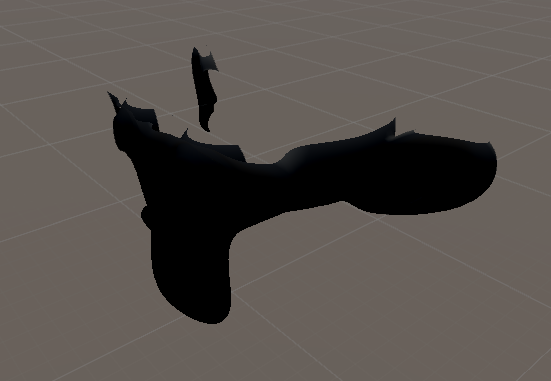Now we can simply see through the upper part of the model. With this done, we don’t need the visualisation anymore and can use colors we use usually again.

``````//the surface shader function which sets parameters the lighting function then uses
void surf (Input i, inout SurfaceOutputStandard o) {
//calculate signed distance to plane
float distance = dot(i.worldPos, _Plane.xyz);
distance = distance + _Plane.w;
o.Emission = distance;

//normal color stuff
fixed4 col = tex2D(_MainTex, i.uv_MainTex);
col *= _Color;
o.Albedo = col.rgb;
o.Metallic = _Metallic;
o.Smoothness = _Smoothness;
o.Emission = _Emission;
}
``````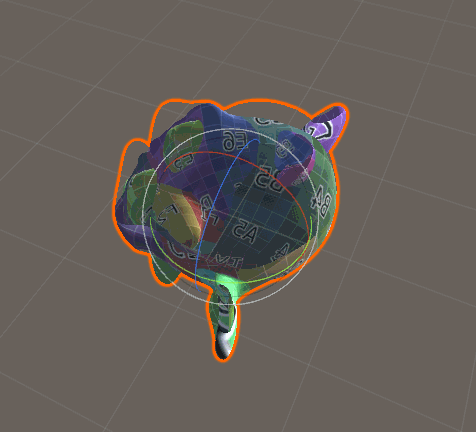## Show Inside

With those changes we can now cut off the model based on a plane, but looking in the hole we created looks weird. Especially concave bodies look like they have small parts of them flying around sometimes. This is because by default we don’t draw the backfaces of models. It’s a optimisation we can make because we assume we won’t see inside the model anyways, but we can simply disable it.

To draw all faces, no matter if they’re pointing towards the camera or away from it, we set the Cull parameter to off at the top of our subshader, outside of the hlsl code.

``````SubShader{
//the material is completely non-transparent and is rendered at the same time as the other opaque geometry
Tags{ "RenderType"="Opaque" "Queue"="Geometry"}

// render faces regardless if they point towards the camera or away from it
Cull Off
``````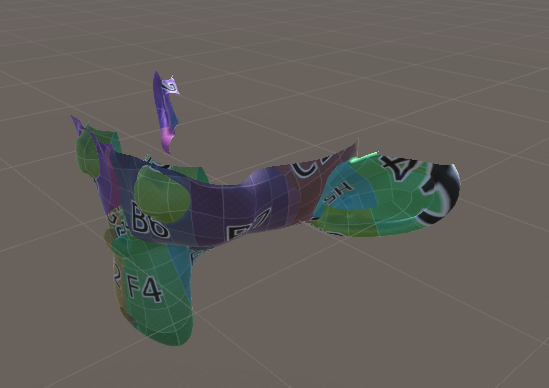Now we can see inside the head, but the normals still point to the outside and we might not want to see the inside of the head. But can detect the difference between the inside surface and outside surface pretty easily so let’s do that.

To get wether we’re rendering a inside or a outside surface we make a new parameter in our input struct and give it the vface attribute. This variable will have a value of 1 one the outside and a value of -1 on the inside.

To use the value for things like linear interpolation I prefer to have it in a 0 to 1 range so I halved it and added 0.5 to it to convert it.

``````//input struct which is automatically filled by unity
struct Input {
float2 uv_MainTex;
float3 worldPos;
float facing : VFACE;
};
``````
``````float facing = i.facing * 0.5 + 0.5;
o.Emission = facing;
``````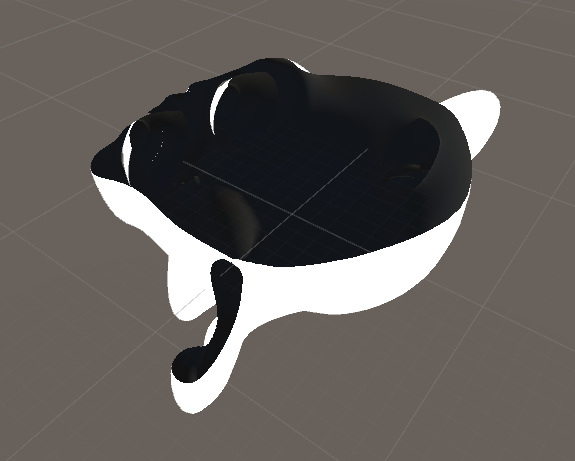Now that we know the difference between the inside and outside faces, we can make the inside it’s own specific color. We lerp to the new color we expose via a property on the emissive channel because the emission is not affected by the wrong normals. We also multiply all other channels with the facing variable to make them black/matte/non-metallic to make the color we can see in the opening as neutral as possible.

``````//show values to edit in inspector
Properties{
_Color ("Tint", Color) = (0, 0, 0, 1)
_MainTex ("Texture", 2D) = "white" {}
_Smoothness ("Smoothness", Range(0, 1)) = 0
_Metallic ("Metalness", Range(0, 1)) = 0
[HDR]_Emission ("Emission", color) = (0,0,0)

[HDR]_CutoffColor("Cutoff Color", Color) = (1,0,0,0)
}
``````
``````    float4 _CutoffColor;
``````
``````float facing = i.facing * 0.5 + 0.5;

//normal color stuff
fixed4 col = tex2D(_MainTex, i.uv_MainTex);
col *= _Color;
o.Albedo = col.rgb * facing;
o.Metallic = _Metallic * facing;
o.Smoothness = _Smoothness * facing;
o.Emission = lerp(_CutoffColor, _Emission, facing);
``````There are still a few artefacts because of golbal illumination, but we can’t fix them without rewriting/removing global illumination and we won’t do that in this tutorial.

## Source

``````Shader "Tutorial/021_Clipping_Plane"{
//show values to edit in inspector
Properties{
_Color ("Tint", Color) = (0, 0, 0, 1)
_MainTex ("Texture", 2D) = "white" {}
_Smoothness ("Smoothness", Range(0, 1)) = 0
_Metallic ("Metalness", Range(0, 1)) = 0
[HDR]_Emission ("Emission", color) = (0,0,0)

[HDR]_CutoffColor("Cutoff Color", Color) = (1,0,0,0)
}

//the material is completely non-transparent and is rendered at the same time as the other opaque geometry
Tags{ "RenderType"="Opaque" "Queue"="Geometry"}

// render faces regardless if they point towards the camera or away from it
Cull Off

CGPROGRAM
//the shader is a surface shader, meaning that it will be extended by unity in the background
//to have fancy lighting and other features
//our surface shader function is called surf and we use our custom lighting model
#pragma target 3.0

sampler2D _MainTex;
fixed4 _Color;

half _Smoothness;
half _Metallic;
half3 _Emission;

float4 _Plane;

float4 _CutoffColor;

//input struct which is automatically filled by unity
struct Input {
float2 uv_MainTex;
float3 worldPos;
float facing : VFACE;
};

//the surface shader function which sets parameters the lighting function then uses
void surf (Input i, inout SurfaceOutputStandard o) {
//calculate signed distance to plane
float distance = dot(i.worldPos, _Plane.xyz);
distance = distance + _Plane.w;
clip(-distance);

float facing = i.facing * 0.5 + 0.5;

//normal color stuff
fixed4 col = tex2D(_MainTex, i.uv_MainTex);
col *= _Color;
o.Albedo = col.rgb * facing;
o.Metallic = _Metallic * facing;
o.Smoothness = _Smoothness * facing;
o.Emission = lerp(_CutoffColor, _Emission, facing);
}
ENDCG
}